Electron. J. Diff. Eqns., Vol. 2000(2000), No. 01, pp. 1-26.

### Exact multiplicity results for quasilinear boundary-value problems with cubic-like nonlinearities

We consider the boundary-value problem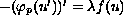in (0,1)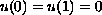where p> 1,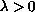and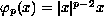. The nonlinearity f is cubic-like with three distinct roots 0=a < b< c. By means of a quadrature method, we provide the exact number of solutions for all. This way we extend a recent result, for p=2, by Korman et al.  to the general case p>1. We shall prove that when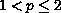the structure of the solution set is exactly the same as that studied in the case p=2 by Korman et al. , and strictly different in the case p>2.

An addendum  was attached on May 3, 2000. There it is shown that Possibility B of Theorem 2.4 and Possibility D of Theorem 2.5 never happens. See last page of this article.

Submitted May 26, 1999. Revised October 1, 1999. Published January 1, 2000.
Math Subject Classifications: 34B15.
Key Words: One dimensional p-Laplacian, multiplicity results, time-maps.

Show me the PDF file (119K), TEX file, and other files for this article.Idris Addou USTHB, Institut de Mathematiques El-Alia, B.P. no. 32 Bab-Ezzouar 16111, Alger, Algerie. e-mail: idrisaddou@hotmail.com